# Example 3: Search engines and PSM filtering¶

In this example we are going to parse the output of several search engines and see what we can do with it using pyteomics. We are going to need all of the extra dependencies of pyteomics: lxml, numpy, matplotlib and pandas.

The files used in this example can be downloaded from http://pubdata.theorchromo.ru/pyteomics_filtering_example/. The directory contains output files from several search engines: X!Tandem, MS Amanda, Morpheus, MS-GF+. All search engines were fed the same spectra. The results do not serve for performance comparison of these search engines, but for illustration of data handling with pyteomics.

The data set was kindly provided by Simion Kreimer at Northeastern University in Boston.

In :
%pylab --no-import-all inline
import seaborn as sb # makes the plots look nicer just by sitting there. Optional.
from pyteomics import tandem, pepxml, mzid, auxiliary as aux, pylab_aux as pa
import pandas as pd

Populating the interactive namespace from numpy and matplotlib


Now, let's read an X!Tandem file using tandem.read() and build a histogram of retention times for identified spectra. Then, let's do the same for spectra passing the 1% FDR threshold, by using tandem.filter().

In :
with tandem.read('example.t.xml') as tf:
h = pylab.hist([psm['rt'] / 60 for psm in tf], bins=25, label='All IDs')
with tandem.filter('example.t.xml', fdr=0.01, full_output=False) as ftf:
pylab.hist([psm['rt'] / 60 for psm in ftf], bins=h, label='1% FDR')
pylab.xlabel('RT, min')
pylab.legend()

Out:
<matplotlib.legend.Legend at 0x7ff900cbec88>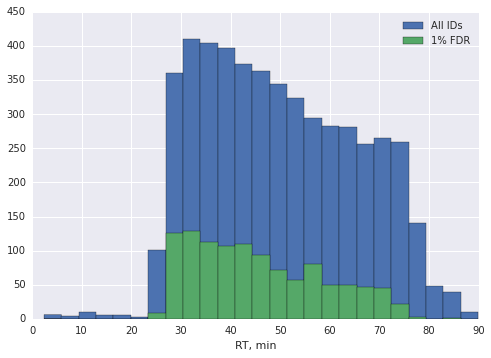The filter() functions can be used in the same way as read() functions (as a context manager). That is done by specifying full_output=False. The benefit of this is lower memory usage when parsing XML files. The downside, however, is that parsing occurs twice, affecting the execution time. That's why the default behavior of filter() is simply to return an array of PSMs:

In :
%%time
ftf = tandem.filter('example.t.xml', fdr=0.01)
rts = [psm['rt'] / 60 for psm in ftf]

CPU times: user 14.9 s, sys: 90 ms, total: 15 s
Wall time: 15 s

In :
%%time
with tandem.filter('example.t.xml', fdr=0.01, full_output=False) as ftf:
rts = [psm['rt'] / 60 for psm in ftf]

CPU times: user 24.4 s, sys: 20 ms, total: 24.4 s
Wall time: 24.4 s


Another way would be to build a pandas.DataFrame object and access its columns. This enables you to write concise and efficient code. The tandem and pepxml modules define the functions DataFrame() and filter_df(), analogous to read() and filter().

In :
%%time
ftf = tandem.filter_df('example.t.xml', fdr=0.01)
rts = ftf['rt']

CPU times: user 14.9 s, sys: 16.7 ms, total: 14.9 s
Wall time: 14.9 s


A function closely related to filter(), qvalues(), calculates q-values for all PSMs in a set. filter() uses it internally to determine where to set the threshold.

In :
q1 = pepxml.qvalues('example.pep.xml', read_schema=False,
key=lambda x: x['search_hit']['search_score']['Morpheus Score'], reverse=True)
q2 = tandem.qvalues('example.t.xml')

pa.plot_qvalue_curve(q1['q'], label='Morpheus')
pa.plot_qvalue_curve(q2['q'], label='X!Tandem')
pylab.legend()

Out:
<matplotlib.legend.Legend at 0x7ff8ffcf1710>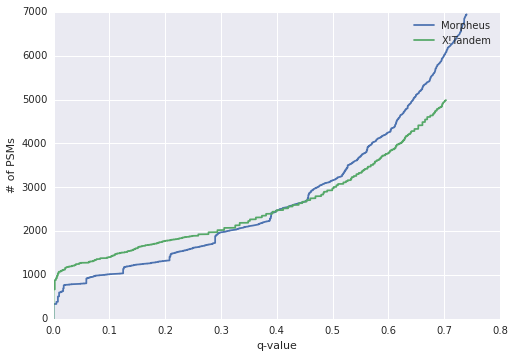The mzid module also provides qvalues() and filter() functions.

In :
msgf = mzid.filter('example.mzid', retrieve_refs=True,
key=lambda x: x['SpectrumIdentificationItem']['MS-GF:EValue'], fdr=0.01)
pylab.hist([psm['SpectrumIdentificationItem']['chargeState'] for psm in msgf], bins=np.arange(5), align='left')
pylab.xticks(np.linspace(0, 4, 5))
pylab.xlabel('charge state')

Out:
<matplotlib.text.Text at 0x7ff900a8d240>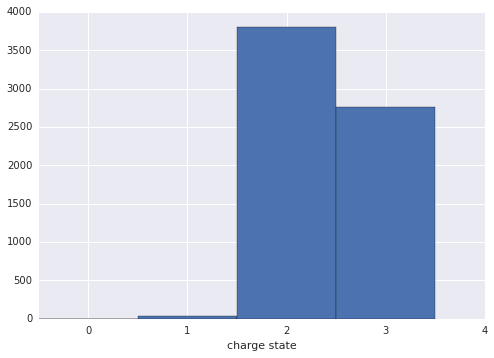pyteomics.auxiliary provides generic functions qvalues() and filter() that you can use on any objects representing PSMs. They accept iterables of PSMs (lists, iterators, arrays, DataFrames).

The q-values are calculated using standard target-decoy approach or using posterior error probabilities, if they are provided. With TDA, you need to provide a way to determine the score of a PSM, and whether it is decoy or not. You can do that by passing a function or an array of values. With structured arrays and DataFrames, however, you can simply provide a column / field label. Let's see how easy it is to use filter() on the output of Morpheus and MS Amanda using pandas.

In :
# read the files into DataFrames

In :
# filter the Morpheus DataFrame
morph_filt = aux.filter(morpheus, fdr=0.01, key='Morpheus Score', reverse=True, is_decoy='Decoy?')

In :
# do some analysis
morph_filt.plot(x='Retention Time (minutes)', y='Precursor Mass (Da)', kind='scatter')

Out:
<matplotlib.axes._subplots.AxesSubplot at 0x7ff900a86da0>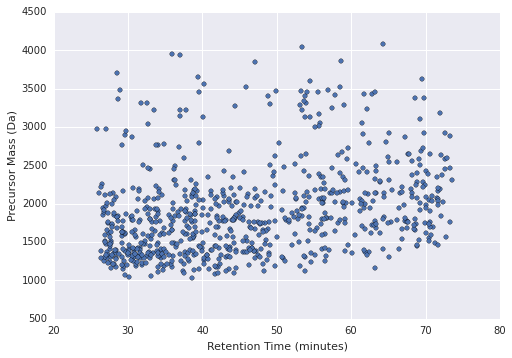In :
# MS Amanda files don't have a "decoy" column, so let's create one and then use it
amanda['isDecoy'] = amanda['Protein Accessions'].str.split(';').apply(
lambda x: all(p.startswith('DECOY') for p in x))
amanda_filt = aux.filter(amanda[amanda['Rank'] == 1], key='Weighted Probability', is_decoy='isDecoy', fdr=0.01)


Wasn't that easy? It is also efficient, because we don't need to parse XML, and the calculations are vectorized by pandas.

Now we have our filtered DataFrames. We can do something using the awesome pandas functionality. For example, let's convert these filtered PSM lists to peptide lists.

In :
amanda_pep = amanda_filt.sort('Weighted Probability').groupby('Sequence').first()
morph_pep = morph_filt.sort('Q-Value (%)').groupby('Base Peptide Sequence').first()


That's it! Now, why don't we look at peptides present in both lists? Peace of cake for pandas, too:

In :
inter = amanda_pep.join(morph_pep, how='inner', lsuffix='[amanda]', rsuffix='[morpheus]')


inter is now a DataFrame with all information from both tables (and that's a lot).

In :
inter.plot('Amanda Score', 'Morpheus Score', kind='hexbin', gridsize=10, sharex=False)

Out:
<matplotlib.axes._subplots.AxesSubplot at 0x7ff8fdc5b4a8>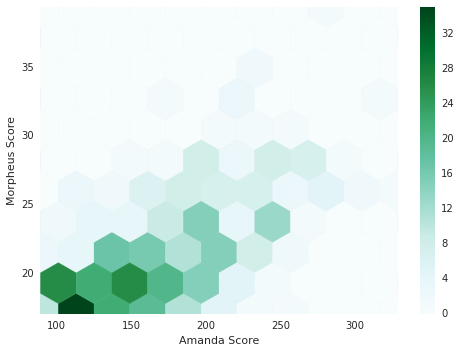We will conclude this example with a reminder that if you have a piece of software that reports posterior error probabilities (PEPs), qvalues() and filter() can use them instead of relying on TDA. The usage is similar: you just provide a pep parameter instead of is_decoy. key is not needed in this case, too. Complete information about possible parameters can be found in the API docs.